# Updated SPSS Chart Template (V26) and Chart Notes

So I was helping someone out the other day with SPSS chart templates, and figured it would be a good opportunity to update mine. I have a new template version for V26, V26_ChartStyle.sgt. I also have some code to illustrate the template, plus a few random SPSS charting tips along the way.

For notes about chart templates, I have written about it previously, and Ruben has a nice tutorial on his site as well. For those not familiar, SPSS chart templates specify the default looks of the chart, very similar to CSS for HTML. So for example, if you want your labels to be a particular font, or you want the background for the chart to be light grey, or you want the gridlines to be dashed, are all examples you can specify in a chart template.

It is plain text XML file under the hood – unfortunately there is not any official documentation about what is valid from IBM SPSS that I am aware of, so to amend it just takes trial and error. So in case anyone from SPSS pays attention here, if you have other docs to note let me know!

Below I will walk through my updated template and various charts to illustrate the components of it.

# Walkthrough Template Example

So first to start out, if you want to specify a new template, you can either do it via the GUI (Edit -> Options -> Charts Tab), or via syntax such as `SET CTEMPLATE='data\template.sgt'`. Here I make some fake data to illustrate various chart types.

``````SET SEED 10.
INPUT PROGRAM.
LOOP Id = 1 TO 20.
END CASE.
END LOOP.
END FILE.
END INPUT PROGRAM.
DATASET NAME Test.
COMPUTE Group = TRUNC(RV.UNIFORM(1,7)).
COMPUTE Pair = RV.BERNOULLI(0.5).
COMPUTE X = RV.UNIFORM(0,1).
COMPUTE Y = RV.UNIFORM(0,1).
COMPUTE Time = MOD(Id-1,10) + 1.
COMPUTE TGroup = TRUNC((Id-1)/10).
FORMATS Id Group Pair TGroup Time (F2.0) X Y (F2.1).
VALUE LABELS Group
1 'A'
2 'B'
3 'C'
4 'D'
5 'E'
6 'F'
.
VALUE LABELS Pair
0 'Group One'
1 'Group Two'
.
VALUE LABELS TGroup
0 'G1'
1 'G2'
.
EXECUTE.``````

Now to start out, I show off my new color palette using a bar chart. I use a color palette derived from a Van Gogh’s bedroom as my default set. An idea I got from Sidonie Christophe (and I used this palette generator website). I also use a monospace font, Consolas, for all of the text (SPSS pulls from the system fonts, and I am a windows guy). The default font sizes are too small IMO, especially for presentations, so the X/Y axes tick labels are 14pt.

``````*Bar graph to show colors, title, legend.
GGRAPH
/GRAPHDATASET NAME="graphdataset" VARIABLES=Group MEAN(X)[name="MEAN"]
/GRAPHSPEC SOURCE=INLINE.
BEGIN GPL
SOURCE: s=userSource(id("graphdataset"))
DATA: Group=col(source(s), name("Group"), unit.category())
DATA: X=col(source(s), name("MEAN"))
GUIDE: axis(dim(1), label("Group"))
GUIDE: axis(dim(2), label("Mean X"))
GUIDE: legend(aesthetic(aesthetic.color.interior), label("Group"))
GUIDE: text.title(label("Main Title"))
GUIDE: text.subtitle(label("Subtitle"))
SCALE: cat(dim(1), include("1", "2", "3", "4", "5", "6"))
SCALE: linear(dim(2), include(0))
ELEMENT: interval(position(Group*X), shape.interior(shape.square), color.interior(Group))
END GPL.``````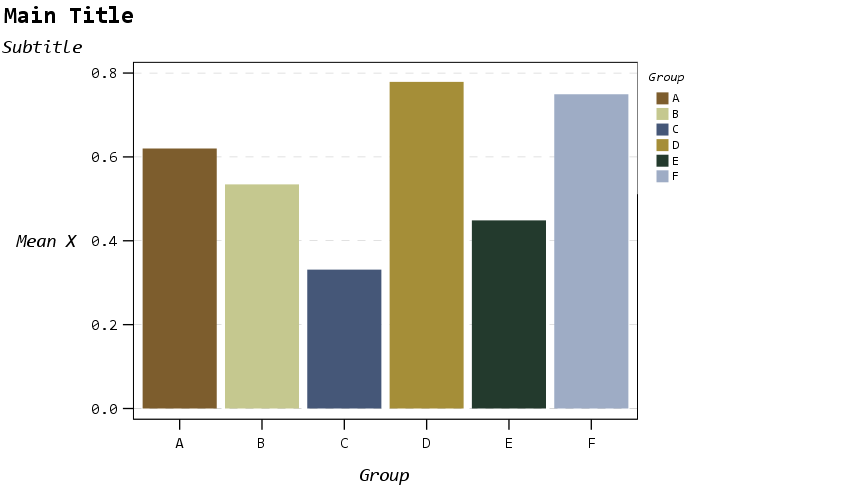The legend I would actually prefer to have an outline box, but it doesn’t behave so nicely when I use a continuous aesthetic and pads too much. I will end the blog post with things I wish I could do with templates and/or GPL. (I wished for documentation to help me with the template for legends for example, but wish I could specify the location of the legend in inline GPL code.)

The next chart shows a scatterplot. One thing I make sure in the template is to not prevent you specifying what you want in inline GPL that is possible. So for example you could specify a default scatterplot of size 12 and the inside is grey, but as far as I can tell that prevents you from changing the color later on. Also I show a trick with using the subtitle to make a Y axis label at the top of the chart, a trick I saw originally from Naomi Robbins. (She actually prefers the text orientation on the side running north/south if you do that approach, but I prevented that in this template, I really dislike having to turn my head to read it!)

``````* Scatterplot, showing Y axis trick with subtitle.
* Would prefer the setStyle subtype="simple" type="scatter" works as default.
GGRAPH
/GRAPHDATASET NAME="graphdataset" VARIABLES=X Y
/GRAPHSPEC SOURCE=INLINE
/FITLINE TOTAL=NO.
BEGIN GPL
SOURCE: s=userSource(id("graphdataset"))
DATA: X=col(source(s), name("X"))
DATA: Y=col(source(s), name("Y"))
GUIDE: axis(dim(1), label("X Label"))
GUIDE: axis(dim(2))
GUIDE: text.subtitle(label("  Y Label"))
ELEMENT: point(position(X*Y), size(size."12"), color.interior(color."bebebe"))
END GPL.``````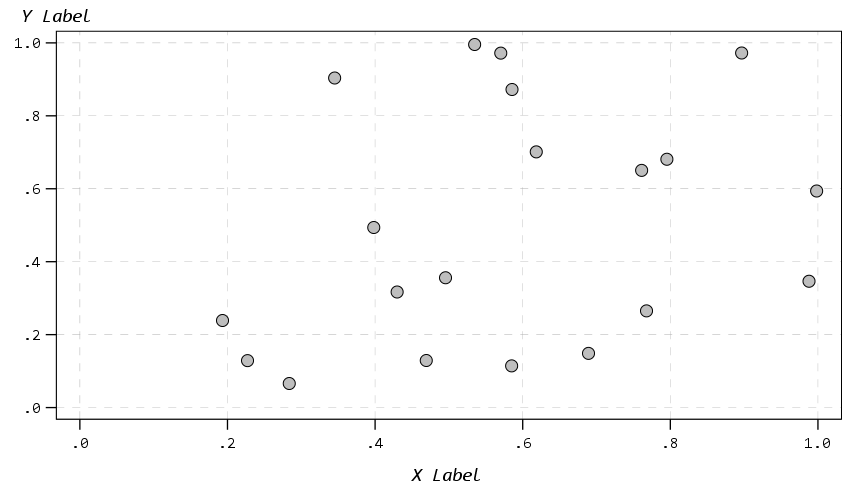The next chart shows off my default data labels. I have the labels positioned at the center point, and also inherit the color from the data element. So one trick I like to use is to use the polygon element to explicitly set the locations of labels (and you can draw the polygons transparent, so you only see the labels in the end). So if you want to put labels at the top of bars (or above a line graph) like Excel does, you can just shift them up a smidge.

``````*Label trick for bar graphs.
GGRAPH
/GRAPHDATASET NAME="graphdataset" VARIABLES=Group COUNT()[name="COUNT"]
/GRAPHSPEC SOURCE=INLINE.
BEGIN GPL
SOURCE: s=userSource(id("graphdataset"))
DATA: Group=col(source(s), name("Group"), unit.category())
DATA: COUNT=col(source(s), name("COUNT"))
TRANS: ext = eval(COUNT + 0.2)
GUIDE: axis(dim(1))
GUIDE: axis(dim(2))
GUIDE: text.subtitle(label("Count"))
SCALE: cat(dim(1), include("1", "2", "3", "4", "5", "6"))
SCALE: linear(dim(2), include(0))
ELEMENT: interval(position(Group*COUNT), color.interior(color."bebebe"))
ELEMENT: polygon(position(Group*ext), label(COUNT), color.interior(color.red),
transparency.interior(transparency."1"), transparency.exterior(transparency."1"))
END GPL.``````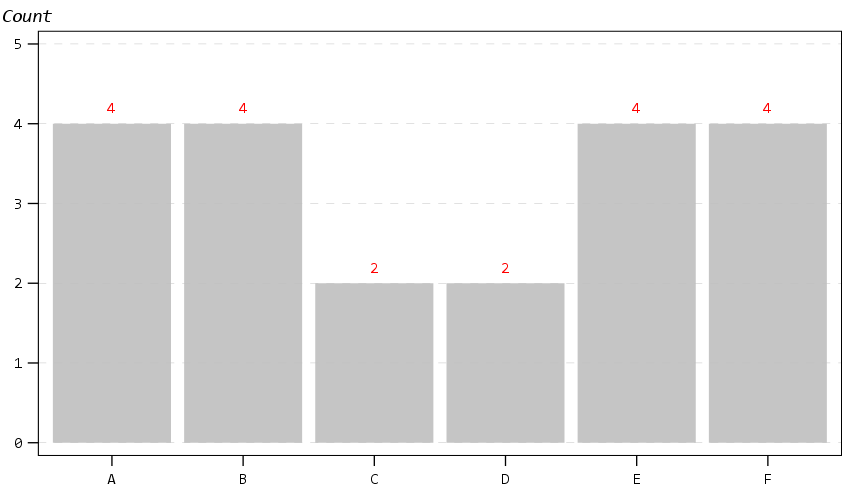Next up is a histogram. SPSS has an annoying habit of publishing a statistics summary frame with mean/standard deviation when you make a histogram. Unfortunately the only solution I have found to this is to still generate the frame, but it is invisible so doesn’t show anything. It still takes up space in the chart area though, so the histogram will be shrunk to be somewhat smaller in width. Also I was unable to find a solution to not print the Frequency numbers with decimals here. (You can use `setTickLabelFormat` to say no decimals, but then that applies to all charts by default. SPSS typically chooses the numeric format from the data, but here since it calculates it inline GPL, there is no way to specify it in advance that I know of.)

``````* Histogram, no summary frame.
* Still not happy with Frequency numbers, stat summary is there but is empty.
GGRAPH
/GRAPHDATASET NAME="graphdataset" VARIABLES=Y
/GRAPHSPEC SOURCE=INLINE.
BEGIN GPL
SOURCE: s=userSource(id("graphdataset"))
DATA: Y=col(source(s), name("Y"))
GUIDE: axis(dim(1), label("Y"))
GUIDE: axis(dim(2), label("Frequency"))
GUIDE: text.title(label("Simple Histogram of Y"))
ELEMENT: interval(position(summary.count(bin.rect(Y, binWidth(0.1), binStart(-0.05) ))),
color.interior(color."bebebe"), color.exterior(color.white),
transparency.interior(transparency."0.35"), transparency.exterior(transparency."0.35"))
END GPL.``````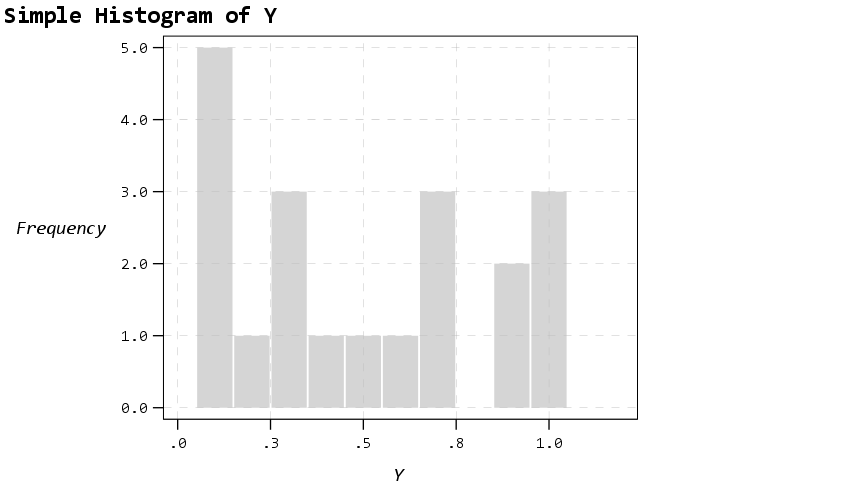The next few charts I will illustrate how SPSS pulls the axes label formats from the data itself. So first I create a few new variables for percentages, dollars, and long values with comma groupings. Additionally a new part of this template is I was able to figure out how to set the text style for small multiple panels (they are ticked like tick marks for X/Y axes). So this illustrates my favorite way to mark panels.

``````* Small multiple columns, illustrating different variable formats.
COMPUTE XPct = X*100.
COMPUTE YDollar = Y * 5000.
COMPUTE YComma = Y * 50000.
*Can also do PCT3.1, DOLLAR5.2, COMMA 4.1, etc.
FORMATS XPct (PCT3) YDollar (DOLLAR4) YComma (COMMA7).
EXECUTE.

GGRAPH
/GRAPHDATASET NAME="graphdataset" VARIABLES=XPct YDollar Pair
/GRAPHSPEC SOURCE=INLINE
/FITLINE TOTAL=NO.
BEGIN GPL
SOURCE: s=userSource(id("graphdataset"))
DATA: X=col(source(s), name("XPct"))
DATA: Y=col(source(s), name("YDollar"))
DATA: Pair=col(source(s), name("Pair"), unit.category())
GUIDE: axis(dim(1), label("X Label"))
GUIDE: axis(dim(2), label("Y Label"))
GUIDE: axis(dim(3), opposite())
ELEMENT: point(position(X*Y*Pair), size(size."12"), color.interior(color."bebebe"))
END GPL.``````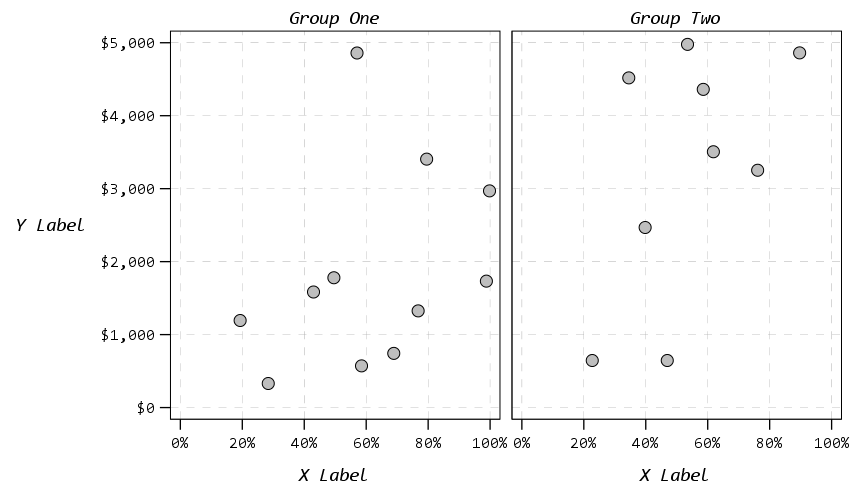That is to make panels in columns, but you can also do it in rows. And again I prevent all the text I can from turning vertical up/down, and force it to write the text horizontally.

``````* Small multiple rows.
GGRAPH
/GRAPHDATASET NAME="graphdataset" VARIABLES=X YComma Pair
/GRAPHSPEC SOURCE=INLINE
/FITLINE TOTAL=NO.
BEGIN GPL
SOURCE: s=userSource(id("graphdataset"))
DATA: X=col(source(s), name("X"))
DATA: Y=col(source(s), name("YComma"))
DATA: Pair=col(source(s), name("Pair"), unit.category())
GUIDE: axis(dim(1), label("X Label"))
GUIDE: axis(dim(2), label("Y Label"))
GUIDE: axis(dim(4), opposite())
ELEMENT: point(position(X*Y*1*Pair))
END GPL.``````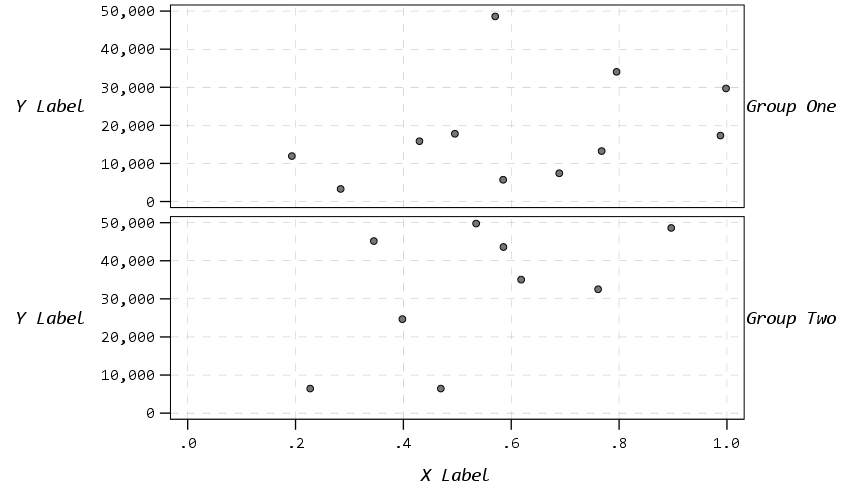And finally if you do wrapped panels and set the facet label to opposite, it puts the grid label on the top of the panel.

``````* Small multiple wrap.
GGRAPH
/GRAPHDATASET NAME="graphdataset" VARIABLES=X Y Group
/GRAPHSPEC SOURCE=INLINE
/FITLINE TOTAL=NO.
BEGIN GPL
SOURCE: s=userSource(id("graphdataset"))
DATA: X=col(source(s), name("X"))
DATA: Y=col(source(s), name("Y"))
DATA: Group=col(source(s), name("Group"), unit.category())
COORD: rect(dim(1,2), wrap())
GUIDE: axis(dim(1))
GUIDE: axis(dim(2))
GUIDE: axis(dim(3), opposite())
ELEMENT: point(position(X*Y*Group))
END GPL.``````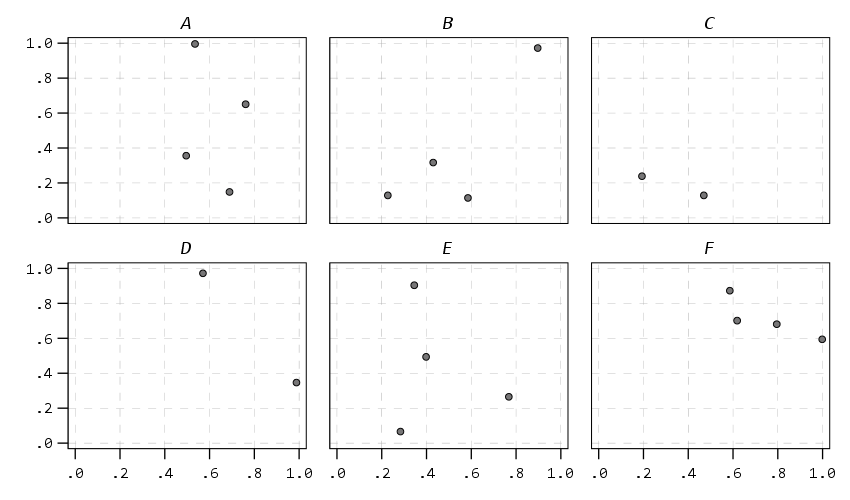Next up I show how my default colors do with line charts, I typically tell people to avoid yellow for lines, but this tan color does alright I think. (And Van Gogh was probably color blind, so it works out well for that.) I have not tested out printing in grey-scale, I don’t think it will work out well for that beyond just the first two colors.

``````* Multiple line chart (long format).
GGRAPH
/GRAPHDATASET NAME="graphdataset" VARIABLES=Time Y TGroup
/GRAPHSPEC SOURCE=INLINE.
BEGIN GPL
SOURCE: s=userSource(id("graphdataset"))
DATA: Time=col(source(s), name("Time"))
DATA: Y=col(source(s), name("Y"))
DATA: TGroup=col(source(s), name("TGroup"), unit.category())
SCALE: linear(dim(1), min(1))
GUIDE: axis(dim(1), delta(1))
GUIDE: axis(dim(2))
GUIDE: legend(aesthetic(aesthetic.color.interior))
GUIDE: legend(aesthetic(aesthetic.size), label("Size Y"))
GUIDE: text.subtitle(label("   Y"))
SCALE: cat(aesthetic(aesthetic.color.interior), include("0", "1"))
ELEMENT: line(position(Time*Y), color.interior(TGroup))
ELEMENT: point(position(Time*Y), shape(TGroup), color.interior(TGroup),
color.exterior(color.white), size(size."10")) )
END GPL.``````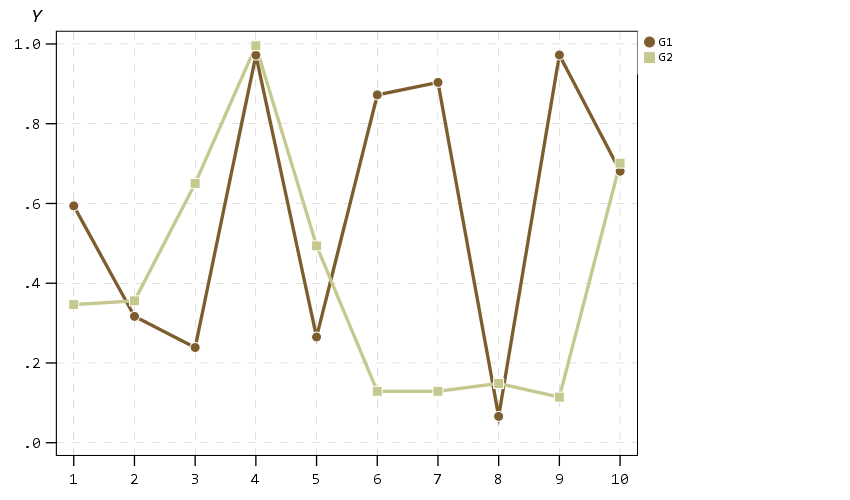A nice approach that forgoes the legend though with line charts is to label the ends of the lines, and I show that below. Also the data above is in long format, and when superimposing points does not quite work out perfectly (G2 should always be above G1, but sometimes the G1 point is above the G2 line). Drawing each individual line in wide format though you can prevent that from happening (but results in more work to write the GPL, need several ELEMENT statements for a single line). I also show how to use splines here, which can sometimes help disentangle the spaghetti lines, but user beware, the interpolated spline values can be misleading (one of the reasons I like superimposing the point markers as well).

``````* Multiple line chart with end labels (wide format).
/BREAK
/LastTime = MAX(Id).
IF Id = LastTime IdLabel = Id.
FORMATS IdLabel (F2.0).
EXECUTE.

GGRAPH
/GRAPHDATASET NAME="graphdataset" VARIABLES=Id X Y IdLabel
MISSING=VARIABLEWISE
/GRAPHSPEC SOURCE=INLINE.
BEGIN GPL
SOURCE: s=userSource(id("graphdataset"))
DATA: Id=col(source(s), name("Id"))
DATA: IdLabel=col(source(s), name("IdLabel"))
DATA: X=col(source(s), name("X"))
DATA: Y=col(source(s), name("Y"))
DATA: TGroup=col(source(s), name("TGroup"), unit.category())
SCALE: linear(dim(1), min(1))
SCALE: linear(dim(2), max(1.08))
GUIDE: axis(dim(1), delta(1))
GUIDE: axis(dim(2))
SCALE: cat(aesthetic(aesthetic.color.interior), include("0", "1"))
ELEMENT: line(position(smooth.spline(Id*Y)), color(color.red))
ELEMENT: line(position(smooth.spline(Id*X)), color(color.blue))
ELEMENT: point(position(Id*Y), color.interior(color.red), color.exterior(color.white),
size(size."9"), shape(shape.circle))
ELEMENT: point(position(Id*X), color.interior(color.blue), color.exterior(color.white),
size(size."8"), shape(shape.square))
ELEMENT: point(position(IdLabel*Y), color(color.red), label("Y"),
transparency.interior(transparency."1"), transparency.exterior(transparency."1"))
ELEMENT: point(position(IdLabel*X), color(color.blue), label("X"),
transparency.interior(transparency."1"), transparency.exterior(transparency."1"))
END GPL.``````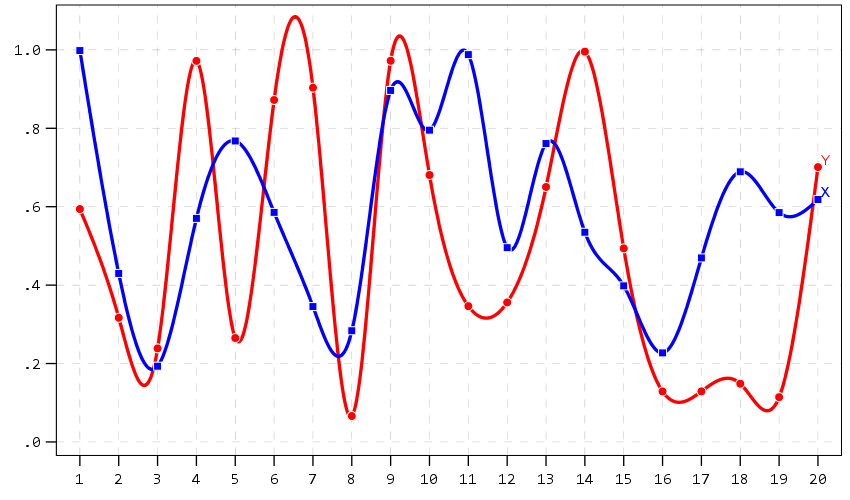A final example I illustrate is an error bar chart, but also include a few different notes about line breaks. You can use a special `\n` character in labels to break lines where you want. Also had a request for labeling the ends of the chart as well. Here I fudge that look by adding in a bunch of white space. This takes trial-and-error to figure out the right number of spaces to include, and can change if the Y axes labels change length, but is the least worst way I can think to do such a task. For error bars and bar graphs, it is also often easier to generate them going vertical, and just use `COORD: transpose()` to make them horizontal if you want.

``````* Error Bar chart.
VALUE LABELS Pair
0 'Group\nOne'
1 'Group\nTwo'
.
GGRAPH
/GRAPHDATASET NAME="graphdataset" VARIABLES=Pair MEANCI(Y, 95)[name="MEAN_Y" LOW="MEAN_Y_LOW"
HIGH="MEAN_Y_HIGH"] MISSING=LISTWISE REPORTMISSING=NO
/GRAPHSPEC SOURCE=INLINE.
BEGIN GPL
SOURCE: s=userSource(id("graphdataset"))
DATA: Pair=col(source(s), name("Pair"), unit.category())
DATA: MEAN_Y=col(source(s), name("MEAN_Y"))
DATA: LOW=col(source(s), name("MEAN_Y_LOW"))
DATA: HIGH=col(source(s), name("MEAN_Y_HIGH"))
COORD: transpose()
GUIDE: axis(dim(1))
GUIDE: axis(dim(2), label("Low Anchor                                                    High Anchor", "\nLine 2"))
GUIDE: text.title(label("Simple Error Bar Mean of Y by Pair\n[Transposed]"))
GUIDE: text.footnote(label("Error Bars: 95% CI"))
SCALE: cat(dim(1), include("0", "1"), reverse() )
SCALE: linear(dim(2), include(0))
ELEMENT: point(position(Pair*MEAN_Y), size(size."12"))
END GPL.``````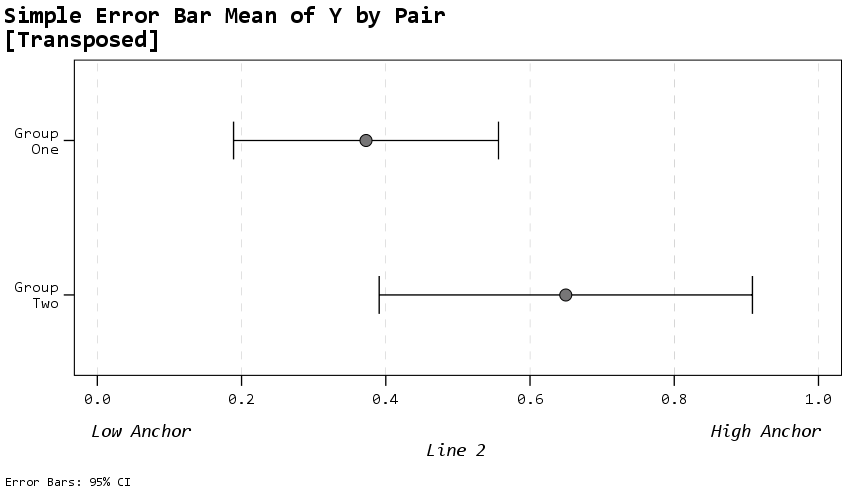Going to back to some scatterplots, I will illustrate a continuous legend example using size of points in a scatterplot. For size elements it typically makes sense to use a square root scale instead of a linear one (SPSS default power scale is to `x^0.5`, so a square root). If you go to edit the chart and click on the legend, you will see here what I mean by the excessive padding of white space at the bottom. (Also wish you could control the breaks that are shown in inline GPL, breaks for non-linear scales though are no doubt tricky.)

``````* Continuous legend example.
GGRAPH
/GRAPHDATASET NAME="graphdataset" VARIABLES=X Y
/GRAPHSPEC SOURCE=INLINE
/FITLINE TOTAL=NO.
BEGIN GPL
SOURCE: s=userSource(id("graphdataset"))
DATA: X=col(source(s), name("X"))
DATA: Y=col(source(s), name("Y"))
GUIDE: axis(dim(1), label("X Label"))
GUIDE: axis(dim(2))
GUIDE: text.subtitle(label("  Y Label"))
GUIDE: legend(aesthetic(aesthetic.size), label("SizeLab"))
SCALE: linear(dim(2), max(1.05))
SCALE: pow(aesthetic(aesthetic.size), aestheticMinimum(size."6px"),
aestheticMaximum(size."30px"), min(0), max(1))
ELEMENT: point(position(X*Y), size(Y), color.interior(color."bebebe"))
END GPL.``````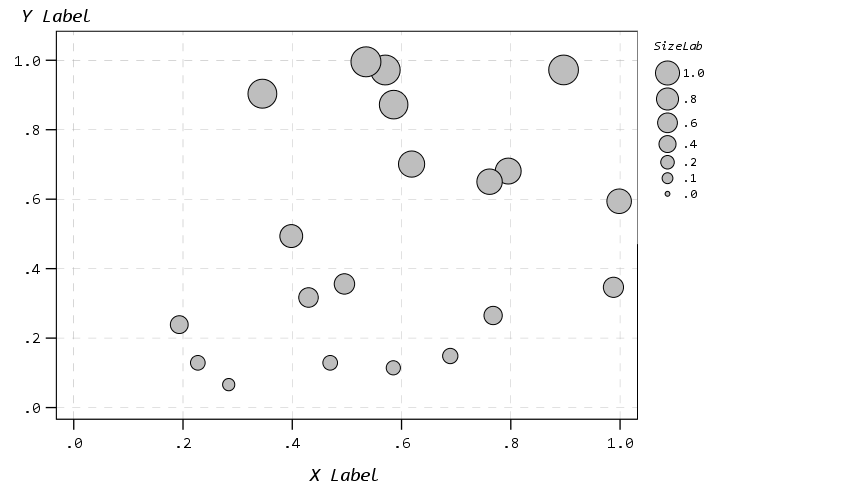And you can also do multiple legends as well. SPSS does a good job blending them together in this example, but to label the group you need to figure out which hierarchy wins first I guess.

``````* Multiple legend example.
GGRAPH
/GRAPHDATASET NAME="graphdataset" VARIABLES=X Y Pair
/GRAPHSPEC SOURCE=INLINE
/FITLINE TOTAL=NO.
BEGIN GPL
SOURCE: s=userSource(id("graphdataset"))
DATA: X=col(source(s), name("X"))
DATA: Y=col(source(s), name("Y"))
DATA: Pair=col(source(s), name("Pair"), unit.category())
GUIDE: axis(dim(1), label("X Label"))
GUIDE: axis(dim(2))
GUIDE: text.subtitle(label("  Y Label"))
GUIDE: legend(aesthetic(aesthetic.color.interior), label("Color/Shape Lab"))
GUIDE: legend(aesthetic(aesthetic.size), label("Size & Trans. Lab"))
SCALE: linear(dim(2), max(1.05))
SCALE: linear(aesthetic(aesthetic.size), aestheticMinimum(size."6px"),
aestheticMaximum(size."30px"), min(0), max(1))
SCALE: linear(aesthetic(aesthetic.transparency.interior), aestheticMinimum(transparency."0"),
aestheticMaximum(transparency."0.6"), min(0), max(1))
ELEMENT: point(position(X*Y), transparency.interior(Y),
shape(Pair), size(Y), color.interior(Pair))
END GPL.``````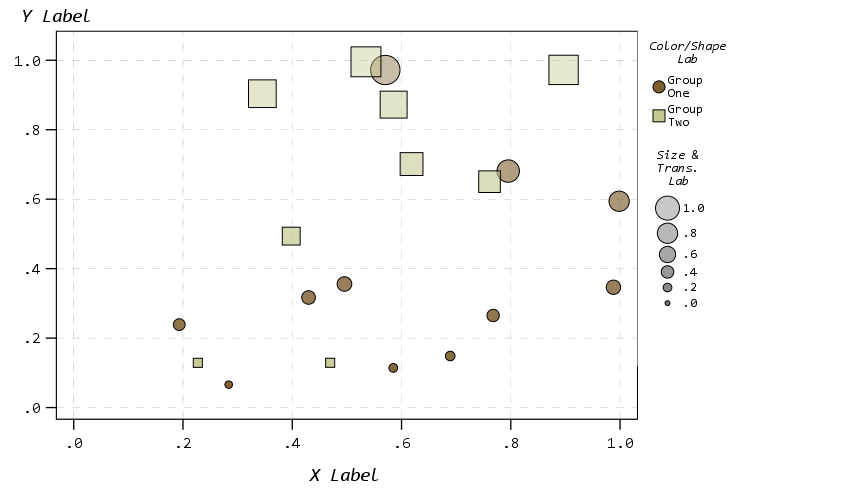But that is not too shabby for just out of the box and no post-hoc editing.

# Wishes for GPL and Templates

So I have put in my comments on things I wish I could do via chart templates. For a recap some documentation on what is possible, turning off the summary statistics for histograms entirely (not just making it invisible), padding for continuous legends, and making the setStyle defaults for the various charts styleOnly are a few examples. And then there are some parts I wish you could do in inline GPL, such as setting the location of the legend. Also I would not mind having access to any of these elements in inline GPL as well, such as say setting the number format in a `GUIDE` statement I think would make sense. (I have no idea how hard/easy these things though are under the hood.) But no documentation for templates is a real hassle when trying to edit your own.

Do y’all have any other suggestions for a chart template? Other default charts I should check out to see how my template does? Other suggested color scales? Let me know your thoughts!